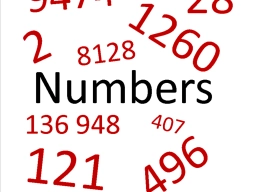# Subtract 80730

I think a number. If I multiply it by three and then subtract 120, I get the number 900. What is that number?

x =  340

### Step-by-step explanation:Did you find an error or inaccuracy? Feel free to write us. Thank you!

Tips for related online calculators
Do you have a linear equation or system of equations and looking for its solution? Or do you have a quadratic equation?